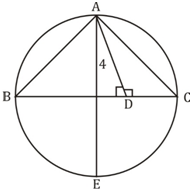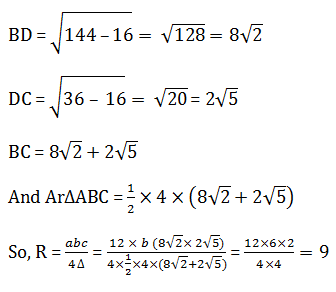# SSC Quantitative Aptitude Questions (Day-67)

Dear Aspirants, you can find the Quantitative Aptitude questions with detailed explanations for the SSC exams. Nowadays the competitive level of the exam has been increasing consistently. Due to the great demand for the government job, the level of the toughness reached greater. Candidates have to enhance the preparation process in order to drive in the right path. It doesn’t need to clear the prescribed cutoff. You must have to score good marks more than the cut off marks to get into the final provisional list. Here we have updating the Quantitative Aptitude questions with detailed explanations on a daily basis. You can practice with us and measure your level of preparation. According to that you can sculpt yourself in a proper way. SSC aspirants kindly make use of it and grab your success in your career.

Start Quiz

1) If (1/x) + (1/y) + (1/z) = 0 and x + y + z = 7, then what is the value of x3 + y3 + z3 – 3xyz?

(a) 49

(b) 343

(c) 1029

(d) 2401

2) If x + (1/x) = 5, then what is the value of x6 + (1/x6)?

(a) 623

(b) 627

(c) 12098

(d) 12102

3)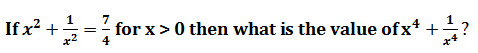(a) 1

(b) 17/16

(c) 15/16

(d) 51/16

4) If
x + 1/x = -2, then what is the value of 1 + x3+x6?

(a) 0

(b) 1

(c) 2

(d) –1

5) What is the simplified value of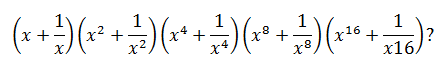(a)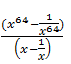(b)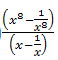(c)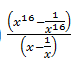(d)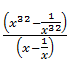6) In ΔABC,
A :B : C = 5 : 4 : 1. What is the value (in degrees) of B + C?

(a) 50

(b) 70

(c) 90

(d) 100

7) If O is the circum-center in ΔPQR and
QOR = 40°, then what is the value (in degrees) of QPR?

(a) 40

(b) 60

(c) 80

(d) 20

8) In ΔDEF,
EFD = 90°, DF = 7 cm and EF = 24. What is the radius (in cm) of the circum-circle of ΔDEF?

(a) 26

(b) 12.5

(c) 13

(d) 25

9) In ΔPQR, PQ = PR and the value of
QPR is equal to half of external angle at R. What is the value (in degrees) of QPR?

(a) 30

(b) 45

(c) 60

(d) 75

10) In the given figure, ABC is a triangle in which, AB = 12 cm, AC = 6 cm and altitude AD = 4 cm. If AE is the diameter of the circum-circle, then what is the length (in cm) of circum-radius?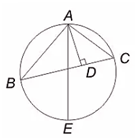(a) 6

(b) 8

(c) 9

(d) 10

xy + yz + zx = 0.                (i)

And x2 + y2 + z2 + 2 (xy + yz + zx) = 49

⟹ x2 + y2 + z2 = 49.                     (ii)

So,

x3 + y2 + z2 – 3xyz. = (x + y + z) [x2 + y2 + z2 – xy – yz –zx].

= 7 [49 – 0] = 343.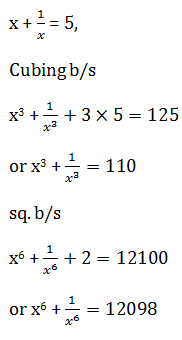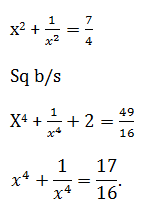x + 1/x = –2

⟹ x = –1.

So, 1 + x3 + x6 = 1 – 1 + 1 = 1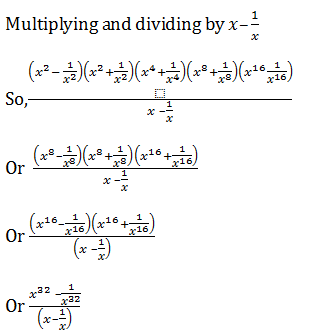10x = 180°

⟹ x = 18°

∴∠B + C = 5 × 18°  = 90°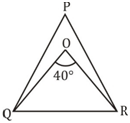∠QPR = ½ × ∠QOR = 20°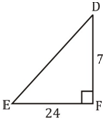So, Circumradius = 25/2  = 12.5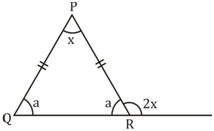2a + x = 180°

And a + 2x = 180°

⟹ 3x = 180°

Or x = 60°